# Maths Video Lesson 2 Revision eNotes Worksheet and Solutions3rd Year Worksheet 2 and Solutions 4

Example 1 & Example 2 4

Example 3 & Example 4 4

Example 5 & Example 6 5

Example 7 5

Example 8 & Example 9 5

Example 12 & Example 13 & Example 14 6

All revision notes have been produced by mockness ltd for mocks.ie.

Email: info@mocks.ie

All rights reserved; no part of this publication may be reproduced, stored in a retrieval system or transmitted, in any form or by any means, electronic, mechanical, photocopying, recording, reprinting, or otherwise without either the prior written permission of Mocks.ie or a license permitting copying in the Republic of Ireland and United Kingdom issued by the copyright licensing Agency.

# 3rd Year Worksheet 2 and Solutions

Now at this stage you should have watched the free Lesson 2 maths tutorial on linear equations and read the mocks.ie lesson 2 revision notes. We are now bringing you a worksheet and solutions.

Example 1 Example 2

Factorise

5ab² 20a²b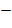= 5ab ( b 4a)Write down the highest common factor.

3x² + 6x

= 3x ( x+2)

## Example 3 Example 4

Factorise

2x (a–2b) – 1 (a-2b)

= (a – 2b)(2x-1)

Factorise

4x² + 3 zy 2 zx 6xy=4x² 6xy + 3zy 2zx=2x ( 2x – 3y)+ z (3y – 2)

=2x ( 2x – 3y) z (3y + 2)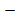= (2x – 3y)(2x – z)

## Example 5 Example 6

Factorise

9a² - 6b²

= 3a ( 3a – 2b²)

Factorise

9a² y ² 1= (3ay)² – (1)²

= (3ay –1)( 3ay –1)

## Example 7

Simplify

(3x+z)(6x2z) – (2y+z)(4y2z)-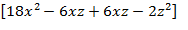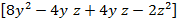= 18x² 2z² –8y² + 2z²= 18x² – 8y²

=2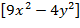=2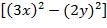= (3x+2y)(3x2y)Example 8 Example 9

Factorise

x² + 11x + 30 30

= (x+5) ( x+b) =1.30

= –1 . –30

= 2.15

= –2. –15

=3.10

= –3 . –10

...